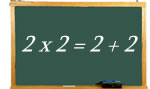Logic puzzles, riddles, math puzzles and brainteasers - pzzls.com

Vandaag is het 2 February 2023

Sort - Most popular on top
Page: << 4 5 6 7 8 >>

# Chess-board - math puzzle

Difficulty:Rating: 3.0/5.0

 How many different squares are located on a chess-board?# 2 x 2 = 2 + 2 - math puzzle

Difficulty:Rating: 2.9/5.0

 Two times two is equal to two and two. But are there more number pairs for which the sum of the numbers is is equal to the product of the numbers? The numbers may be different.# Air trip - math puzzle

Difficulty:Rating: 2.9/5.0

 At the same time two planes depart from JFK international airport. One plane flies exactly in northern direction, while the other one flies exactly to the east. Both planes fly at the same speed and at the same height. Which plane is after one hour the most far away from JFK?